# Supplementary Figure S1 |

 Supplementary Figure S1 |Design look-up maps.a, b Numerically-computed phase distributions of co-polar and cross-polar, respectively, transmission coefficient, as a function of nanohole dimensions, assuming an infinite square array with period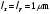with normally-incident, -polarized plane-wave illumination at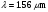. For better visualization, the phase distributions are “unwrapped”.c, d Corresponding magnitude maps. As a reference, a representative equi-magnitude contour (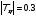) is superposed (dashed-magenta curve) to the cross-polar phase map in panel b. Overall, this results in about 700,000 degrees of freedom. The MUMPS solver is utilized, with default parameters. By varying the nanoholesidelengths and (see Figure 1c) around their resonance values, and illuminating the array with a normally-incident-polarized plane wave at the operational wavelength, we obtain the “look-up” maps shown in Supplementary Figure S1. More specifically, the maps are calculated on a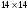grid of (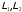) values, and bi-linear interpolation is used for a finer sampling. The transmission coefficients are computed as the scattering parameter pertaining to co- and cross-polarized incident and transmitted zeroth-order modes. It can be observed that, different from the co-polarized case (Supplementary Figure S1a), the phase map pertaining to the cross-polarized component (Supplementary Figure S1b) spans a full range, which is a necessary condition for designing an arbitrary phase profile. In spite of the inherent efficiency limits in the underlying polarization-conversion mechanism, the desired phase span can be attained for moderate values of the transmission-coefficient magnitude, as shown by the reference equi-magnitude contour () superposed on the cross-polar phase map. Based on the look-up maps, we can select the nanohole dimensions so as to synthesize a desired phase distribution of the cross-polar transmission coefficient. More specifically, given the targeted phase-gradient and an even number of elements in a super cell, we initialize the synthesis procedure by choosing the dimensions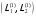of the first element, yielding a phase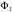of the cross-polar transmission coefficient. We then proceed with the subsequent elements by minimizing the cost function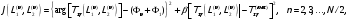22\* MERGEFORMAT (S) where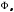denotes the desired phase value,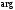is the argument,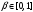is a weight coefficient, and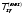is the maximum value of that allows a full phase excursion within the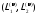-space. Here, and henceforth the principal value of the phase (within the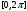range) is assumed. The cost function is minimized via a standard Nelder-Meald (simplex) unconstrained optimization algorithm. The parameters, andare chosen heuristically, via trial-and-error. The remaining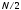elements can be obtained via a rotation of 90° in the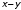plane, which provides a phase-shift on the cross-polar transmission coefficient.1Clearly, the number of free parameters is not sufficient to control both polarization components. Nonetheless, this procedure allows us to obtain a co-polar transmission coefficient with sufficiently uniform magnitude and phase distributions over the designed supercells (see Figure 2). Additional degrees of freedom, e.g., the rotation angle of the nanoholes, could be used to attain better control on both polarization components. The phase gradient can be increased by either reducing , or by increasing the inter-element phase difference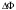. However, some limitations exist, since should be large enough to limit the inter-element coupling effects, andcan be at most equal to in order to guarantee the correct reconstruction of the linear phase profile. The synthesis results summarized in Table 1 implement various combinations of these two parameters.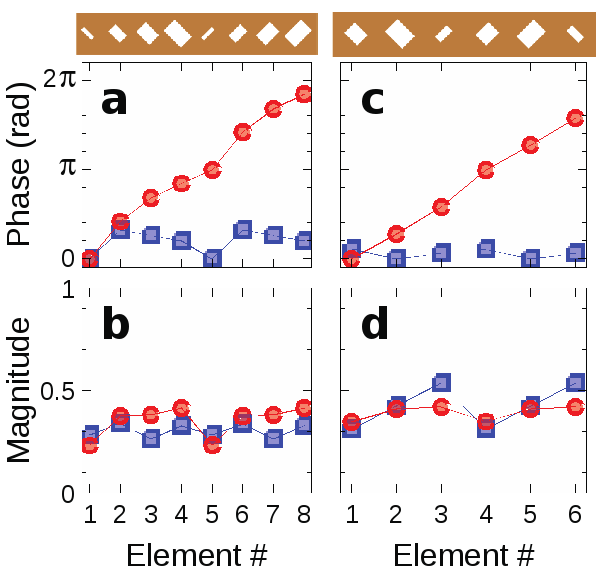Share with your friends: